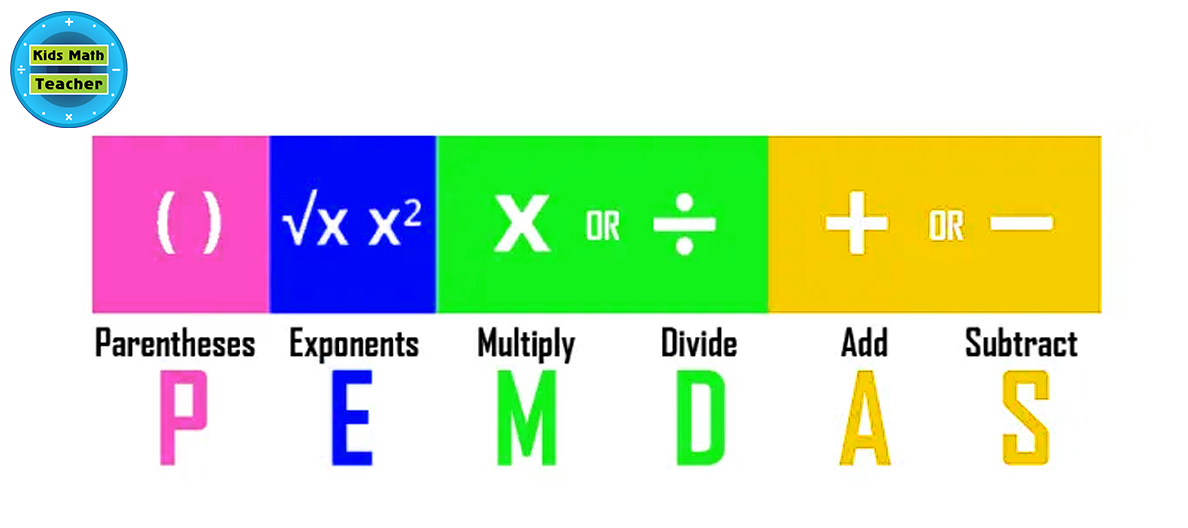+1 424-240-5680

Today we have a visiting contributor, Emma Mooreq. She has a great article to share with us. I have noticed as I had substitute teach in fourth grade classrooms they were not very familiar with this concept. While they might not be working with exponents, it is a very handy rule to know. I still remember it as ‘Please Excuse My Dear Aunt Sally’. Do you remember learning it that way? ~Lucy

___________________________________________

In algebra, the order of operations is frequently used to solve the mathematical expression in a
similar way all over the world by using the PEMDAS rule. This rule is used to avoid the wrong
way of calculations and solve the problems in a well-known order.
In this article, we’ll study how to solve the expressions of mathematics by using the PEMDAS
rule.# What is the PEMDAS rule?

In mathematics, the PEMDAS rule is a rule that expresses the accurate sequence of steps for solving a math expression.

PEMDAS stands for Parentheses, Exponents (power), Multiplication, Division, Addition, & Subtraction.

According to this rule, you have to solve the parentheses of the math expression first, then solve the exponents, after that multiplication & division from left to right, and in the last addition & subtraction from left to right.

If there is more than one similar operation then solve the leftmost operation first and then solve the next one.

## How to solve math expressions by using the PEMDAS rule?

The problems of math expressions can be solved easily either by using the PEMDAS rule or a PEMDAS calculator. Below is an example of a math expression solved by using the PEMDAS rule.

Example

Evaluate 24 x 5 – 142 + 25/5 – (19 + 12) + 3 x (1 + 2) by using the PEMDAS rule.

Solution

Step 1: Write the given math expression.

24 x 5 – 142 + 25/5 – (19 + 12) + 3 x (1 + 2)

Step 2: First of all, solve the parentheses of the given expression.

24 x 5 – 142 + 25/5 – (31) + 3 x (1 + 2)

24 x 5 – 142 + 25/5 – 31 + 3 x (3)

24 x 5 – 142 + 25/5 – 31 + 3 x 3

Step 3: Solve the exponent terms of the expression.

24 x 5 – (14 x 14) + 25/5 – 31 + 3 x 3

24 x 5 – 196 + 25/5 – 31 + 3 x 3

Step 4: Now solve the multiplication & division operations from left to right.

120 – 196 + 25/5 – 31 + 3 x 3

120 – 196 + 5 – 31 + 3 x 3

120 – 196 + 5 – 31 + 9

Step 5: Solve the addition & subtraction operations from left to right.

– 76 + 5 – 31 + 9

– 71 – 31 + 9

– 102 + 9

-93

Hence,

24 x 5 – 142 + 25/5 – (19 + 12) + 3 x (1 + 2) = -93

## Summary

After reading the above article, you can solve any math expression in a correct sequence of steps. The order of operation is not a difficult topic, you have to follow the steps of the PEMDAS rule to find an accurate result.